Related Articles
Sieve of Eratosthenes
• Difficulty Level : Medium
• Last Updated : 31 Mar, 2021

Given a number n, print all primes smaller than or equal to n. It is also given that n is a small number.

Example:

Input : n =10
Output : 2 3 5 7

Input : n = 20
Output: 2 3 5 7 11 13 17 19

The sieve of Eratosthenes is one of the most efficient ways to find all primes smaller than n when n is smaller than 10 million or so (Ref Wiki).

Following is the algorithm to find all the prime numbers less than or equal to a given integer n by the Eratosthene’s method:
When the algorithm terminates, all the numbers in the list that are not marked are prime.

Explanation with Example:
Let us take an example when n = 50. So we need to print all prime numbers smaller than or equal to 50.
We create a list of all numbers from 2 to 50.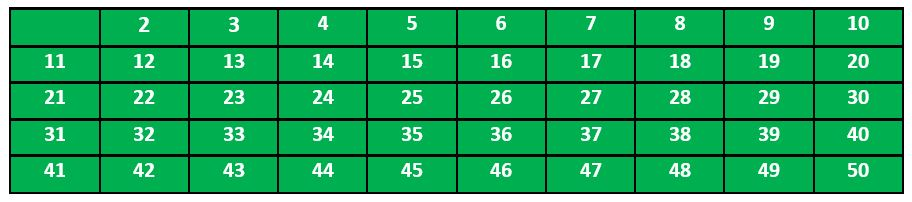According to the algorithm we will mark all the numbers which are divisible by 2 and are greater than or equal to the square of it.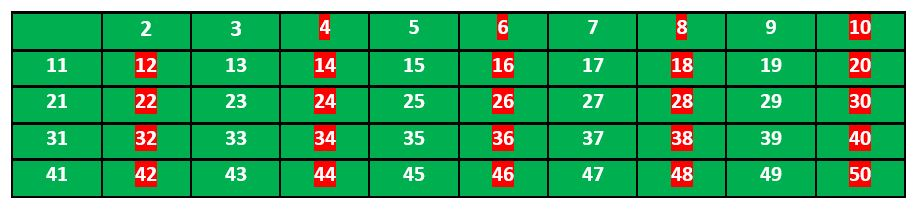Now we move to our next unmarked number 3 and mark all the numbers which are multiples of 3 and are greater than or equal to the square of it.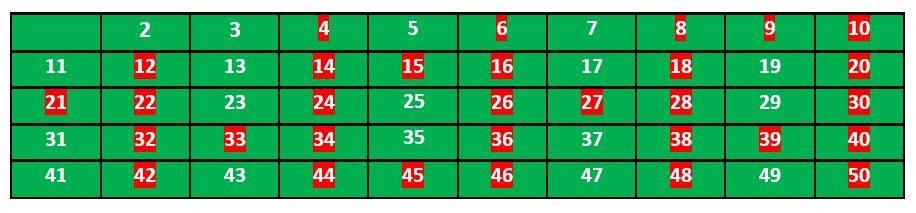We move to our next unmarked number 5 and mark all multiples of 5 and are greater than or equal to the square of it.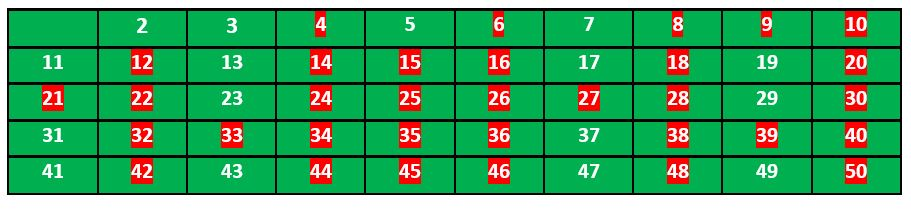We continue this process and our final table will look like below: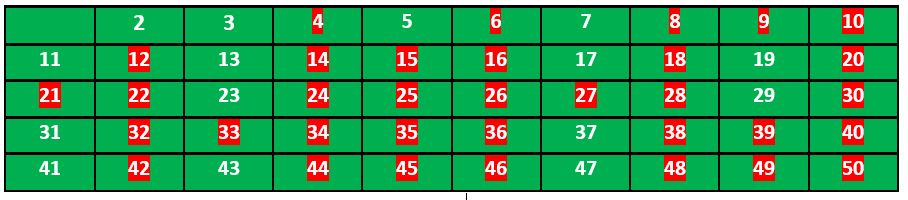So the prime numbers are the unmarked ones: 2, 3, 5, 7, 11, 13, 17, 19, 23, 29, 31, 37, 41, 43, 47.
Thanks to Krishan Kumar for providing above explanation.
Implementation:
Following is the implementation of the above algorithm. In the following implementation, a boolean array arr[] of size n is used to mark multiples of prime numbers.

## C++

 `// C++ program to print all primes``// smaller than or equal to``// n using Sieve of Eratosthenes``#include ``using` `namespace` `std;` `void` `SieveOfEratosthenes(``int` `n)``{``    ``// Create a boolean array``    ``// "prime[0..n]" and initialize``    ``// all entries it as true.``    ``// A value in prime[i] will``    ``// finally be false if i is``    ``// Not a prime, else true.``    ``bool` `prime[n + 1];``    ``memset``(prime, ``true``, ``sizeof``(prime));` `    ``for` `(``int` `p = 2; p * p <= n; p++)``    ``{``        ``// If prime[p] is not changed,``        ``// then it is a prime``        ``if` `(prime[p] == ``true``)``        ``{``            ``// Update all multiples``            ``// of p greater than or``            ``// equal to the square of it``            ``// numbers which are multiple``            ``// of p and are less than p^2``            ``// are already been marked.``            ``for` `(``int` `i = p * p; i <= n; i += p)``                ``prime[i] = ``false``;``        ``}``    ``}` `    ``// Print all prime numbers``    ``for` `(``int` `p = 2; p <= n; p++)``        ``if` `(prime[p])``            ``cout << p << ``" "``;``}` `// Driver Code``int` `main()``{``    ``int` `n = 30;``    ``cout << ``"Following are the prime numbers smaller "``         ``<< ``" than or equal to "` `<< n << endl;``    ``SieveOfEratosthenes(n);``    ``return` `0;``}`

## Java

 `// Java program to print all``// primes smaller than or equal to``// n using Sieve of Eratosthenes` `class` `SieveOfEratosthenes {``    ``void` `sieveOfEratosthenes(``int` `n)``    ``{``        ``// Create a boolean array``        ``// "prime[0..n]" and``        ``// initialize all entries``        ``// it as true. A value in``        ``// prime[i] will finally be``        ``// false if i is Not a``        ``// prime, else true.``        ``boolean` `prime[] = ``new` `boolean``[n + ``1``];``        ``for` `(``int` `i = ``0``; i <= n; i++)``            ``prime[i] = ``true``;` `        ``for` `(``int` `p = ``2``; p * p <= n; p++)``        ``{``            ``// If prime[p] is not changed, then it is a``            ``// prime``            ``if` `(prime[p] == ``true``)``            ``{``                ``// Update all multiples of p``                ``for` `(``int` `i = p * p; i <= n; i += p)``                    ``prime[i] = ``false``;``            ``}``        ``}` `        ``// Print all prime numbers``        ``for` `(``int` `i = ``2``; i <= n; i++)``        ``{``            ``if` `(prime[i] == ``true``)``                ``System.out.print(i + ``" "``);``        ``}``    ``}` `    ``// Driver Code``    ``public` `static` `void` `main(String args[])``    ``{``        ``int` `n = ``30``;``        ``System.out.print(``            ``"Following are the prime numbers "``);``        ``System.out.println(``"smaller than or equal to "` `+ n);``        ``SieveOfEratosthenes g = ``new` `SieveOfEratosthenes();``        ``g.sieveOfEratosthenes(n);``    ``}``}` `// This code has been contributed by Amit Khandelwal.`

## Python

 `# Python program to print all``# primes smaller than or equal to``# n using Sieve of Eratosthenes`  `def` `SieveOfEratosthenes(n):` `    ``# Create a boolean array``    ``# "prime[0..n]" and initialize``    ``#  all entries it as true.``    ``# A value in prime[i] will``    ``# finally be false if i is``    ``# Not a prime, else true.``    ``prime ``=` `[``True` `for` `i ``in` `range``(n``+``1``)]``    ``p ``=` `2``    ``while` `(p ``*` `p <``=` `n):` `        ``# If prime[p] is not``        ``# changed, then it is a prime``        ``if` `(prime[p] ``=``=` `True``):` `            ``# Update all multiples of p``            ``for` `i ``in` `range``(p ``*` `p, n``+``1``, p):``                ``prime[i] ``=` `False``        ``p ``+``=` `1` `    ``# Print all prime numbers``    ``for` `p ``in` `range``(``2``, n``+``1``):``        ``if` `prime[p]:``            ``print` `p,`  `# Driver code``if` `__name__ ``=``=` `'__main__'``:``    ``n ``=` `30``    ``print` `"Following are the prime numbers smaller"``,``    ``print` `"than or equal to"``, n``    ``SieveOfEratosthenes(n)`

## C#

 `// C# program to print all primes``// smaller than or equal to n``// using Sieve of Eratosthenes``using` `System;` `namespace` `prime {``public` `class` `GFG {` `    ``public` `static` `void` `SieveOfEratosthenes(``int` `n)``    ``{` `        ``// Create a boolean array``        ``// "prime[0..n]" and``        ``// initialize all entries``        ``// it as true. A value in``        ``// prime[i] will finally be``        ``// false if i is Not a``        ``// prime, else true.` `        ``bool``[] prime = ``new` `bool``[n + 1];` `        ``for` `(``int` `i = 0; i < n; i++)``            ``prime[i] = ``true``;` `        ``for` `(``int` `p = 2; p * p <= n; p++)``        ``{``            ``// If prime[p] is not changed,``            ``// then it is a prime``            ``if` `(prime[p] == ``true``)``            ``{``                ``// Update all multiples of p``                ``for` `(``int` `i = p * p; i <= n; i += p)``                    ``prime[i] = ``false``;``            ``}``        ``}` `        ``// Print all prime numbers``        ``for` `(``int` `i = 2; i <= n; i++)``        ``{``            ``if` `(prime[i] == ``true``)``                ``Console.Write(i + ``" "``);``        ``}``    ``}` `    ``// Driver Code``    ``public` `static` `void` `Main()``    ``{``        ``int` `n = 30;``        ``Console.WriteLine(``            ``"Following are the prime numbers"``);``        ``Console.WriteLine(``"smaller than or equal to "` `+ n);``        ``SieveOfEratosthenes(n);``    ``}``}``}` `// This code is contributed by Sam007.`

## PHP

 ``

## Javascript

 ``
Output
```Following are the prime numbers smaller  than or equal to 30
2 3 5 7 11 13 17 19 23 29 ```

You may also like to see :

Attention reader! Don’t stop learning now. Get hold of all the important mathematical concepts for competitive programming with the Essential Maths for CP Course at a student-friendly price. To complete your preparation from learning a language to DS Algo and many more,  please refer Complete Interview Preparation Course.

My Personal Notes arrow_drop_up